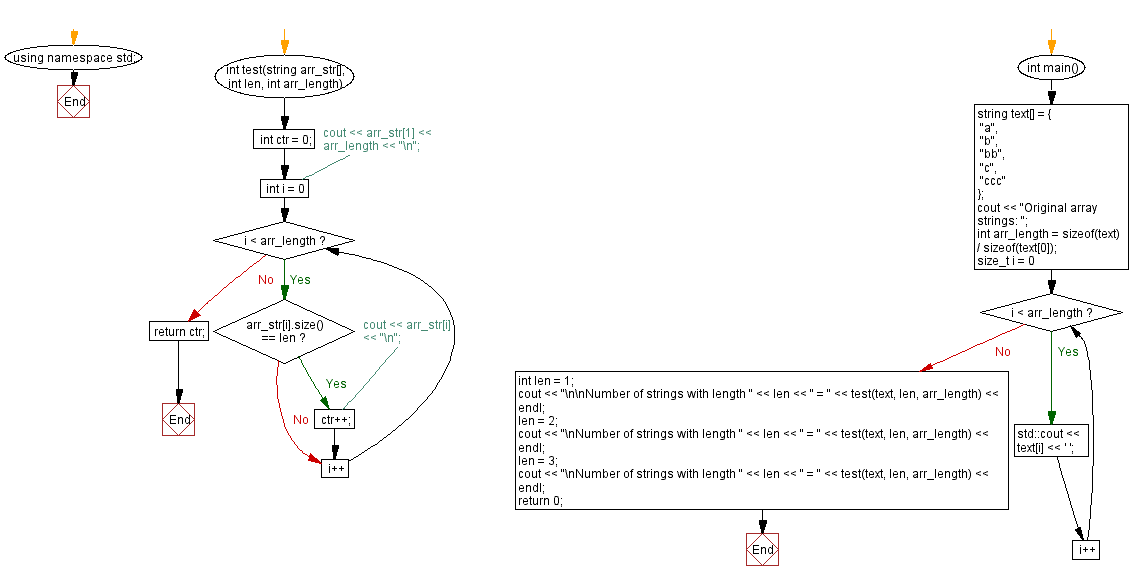﻿ CPP - Number of strings with a given length in an array

# C++ Exercises: Count the number of strings with a given length in an array

## C++ Basic Algorithm: Exercise-119 with Solution

Write a C++ program to count the number of strings with a given length in a given array of strings.

Test Data:
({"a", "b", "bb", "c", "ccc" }, 1) ->3
({"a", "b", "bb", "c", "ccc" }, 2) ->1
({"a", "b", "bb", "c", "ccc" }, 3) ->1

Sample Solution:

C++ Code :

``````#include <iostream>

#include<string.h>
using namespace std;
int test(string arr_str[], int len, int arr_length) {
int ctr = 0;
//  cout << arr_str << arr_length << "\n";
for (int i = 0; i < arr_length; i++) {
if (arr_str[i].size() == len)
// cout << arr_str[i] << "\n";
ctr++;
}
return ctr;
}
int main() {
string text[] = {
"a",
"b",
"bb",
"c",
"ccc"
};
cout << "Original array strings: ";
int arr_length = sizeof(text) / sizeof(text);
for (size_t i = 0; i < arr_length; i++) {
std::cout << text[i] << ' ';
}
int len = 1;
cout << "\n\nNumber of strings with length " << len << " = " << test(text, len, arr_length) << endl;
len = 2;
cout << "\nNumber of strings with length " << len << " = " << test(text, len, arr_length) << endl;
len = 3;
cout << "\nNumber of strings with length " << len << " = " << test(text, len, arr_length) << endl;
return 0;
}
``````

Sample Output:

```Original array strings: a b bb c ccc

Number of strings with length 1 = 3

Number of strings with length 2 = 1

Number of strings with length 3 = 1
```

Flowchart:C++ Code Editor:

What is the difficulty level of this exercise?

﻿

## C++ Programming: Tips of the Day

What is the usefulness of `enable_shared_from_this?

It enables you to get a valid shared_ptr instance to this, when all you have is this. Without it, you would have no way of getting a shared_ptr to this, unless you already had one as a member.

```class Y: public enable_shared_from_this
{
public:

shared_ptr f()
{
return shared_from_this();
}
}

int main()
{
shared_ptr p(new Y);
shared_ptr q = p->f();
assert(p == q);
assert(!(p < q || q < p)); // p and q must share ownership
}
```

The method f() returns a valid shared_ptr, even though it had no member instance. Note that you cannot simply do this:

```class Y: public enable_shared_from_this
{
public:

shared_ptr f()
{
return shared_ptr(this);
}
}
```

The shared pointer that this returned will have a different reference count from the "proper" one, and one of them will end up losing and holding a dangling reference when the object is deleted.

Ref : https://bit.ly/3pwVzzz